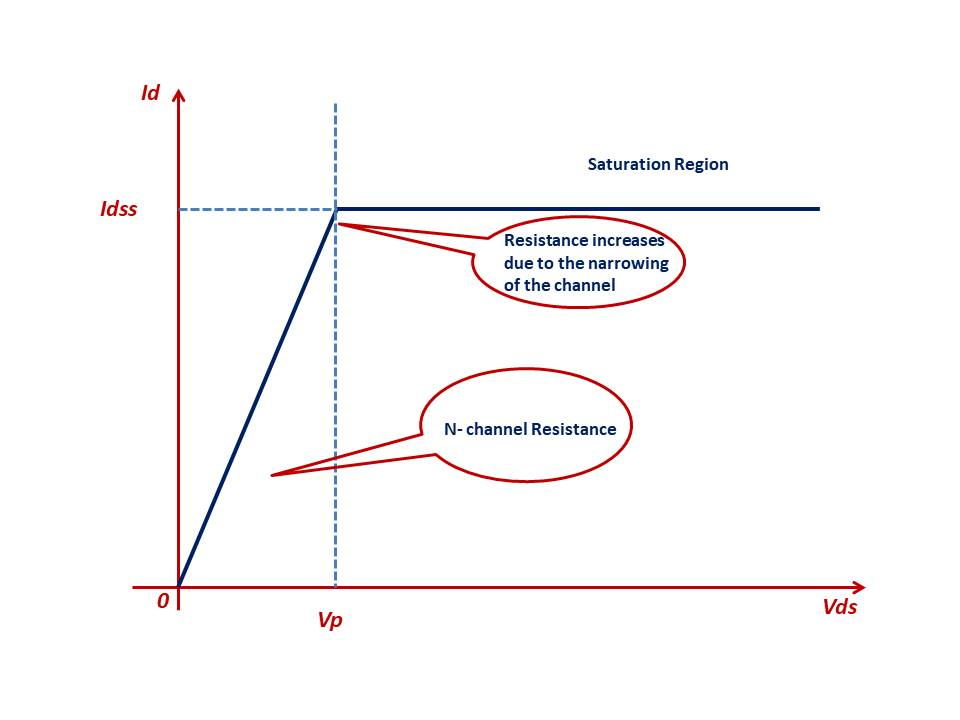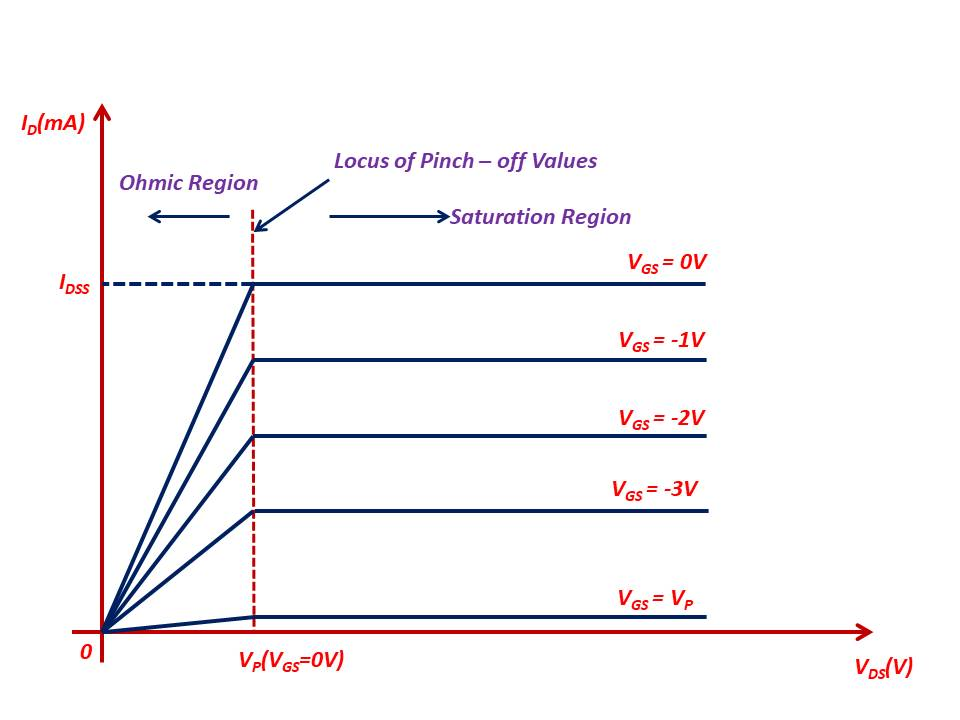### Summary of JFET Operation

Summary of JFET Operation:

Let us summarize the operation of the n-channel JFET studied in the early sections.

Vgs = 0, Vds is positive (Vds>0)

As Vds is applied, the electrons move towards the Drain terminal and Drain Current (Id) starts flowing. The Drain Current Id is limited only by the n-channel resistance present in between the Drain and Source.

As the Drain-Source voltage Vds increases from 0V to a few volts, the Drain current Id will also increase as per Ohm's law as the channel resistance is constant. This region of operation is called the Ohmic region or more popularly as Triode region.

The Figure 7 shows the different regions of operation of the n-channel JFET.Figure 7: Ids vs Vds Characteristics for Vgs = 0 V

As we further increase the voltage Vds it approaches a voltage level called Vp, the depletion region widens, channel width reduces. This results in the reduction of the conduction path i.e the resistance of the channel increases.

As we further increase the voltage Vds, the two depletion regions touch each other as shown in Figure 6 and the n-channel JFET is said to be pinched - off and the value of Vds equals Vp. As the value of the voltage Vds is further increased beyond the pinch off voltage Vp, the two depletion regions which touch each other move across the length of the channel.

As can be seen from the Figure 7 above, the Drain current levels off and remains constant (almost) with further increase in the voltage Vds. The value of this drain current Id is called Idss and it is the maximum current for a given n-channel JFET. The device is said to be operating in the saturation region.

The n-channel JFET under these conditions where Vds > Vp acts like a current source generating a constant current of Idss.

It may be noted here that the maximum Drain current Idss for a JFET is defined for Vgs=0 V and Vds > Vp.

Vgs<0 V

The above section discussed the operation of the n-channel JFET when the Gate - Source voltage VGS is 0V. Here we will discuss the operation of the n-channelJFET when the Vgs is made negative i.e. the voltage applied is Vgs< 0 V.

As a consequence of the Gate Voltage Vgs being made negative, the following will occur:

1. The depletion regions are formed for lower values of the voltage Vds.

2. The device goes into the saturation region for lower values of the voltage Vds.

3. The maximum Drain current Idss also decreases as Vgs is made more and more negative.

As Vgs is made sufficiently negative and reaches the value -Vp, then the drain current almost becomes 0 mA and the device is said to be turned off.

Note 6: The voltage Vp is negative for the n-channel JFET and Vp is positive for p-channel JFET. It also has to be noted that the Gate voltage applied is negative for a n-channel JFET and positive for p-channel JFETFigure 8: n-channel JFET Characteristics showing Ohmic and Saturation Regions

The values shown in the figure 8 are illustrative in nature.
The following points to be noted from the Figure 8:

1. In the Ohmic region the n-channel FET acts as a Voltage Controlled Resistor i.e. the resistance of the device in this region of operation is controlled by the voltage applied at the Gate terminal VGS. Sometimes the Ohmic region may also be called as Voltage Controlled Resistance Region.

2. The slope of the curve which indicates the resistance of the device between Drain and  Source for VDS=VP is a function of VGS.

3. The values of resistance for a particular value of VGS is given by the following equation:​​​​​​​

Where ro is the resistance with VGS=0 V, and rd is the resistance at a particular value of VGS.